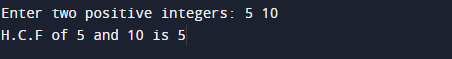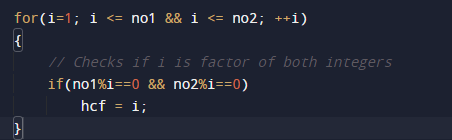C Program to Find HCF of Two Numbers

In this tutorial you will learn about the C Program to Find HCF of Two Numbers and its application with practical example.

In this tutorial, we will learn to create a C program that wil find HCF of two numbers  using C programming.

Prerequisites

Before starting with this tutorial we assume that you are best aware of the following C programming topics:

• Operators.
• General C programming.
• Basic input/output.
• Loop statements
• While loop.
• if else Statement.

What Is HCF?

HCF stands for Highest Common Factor. When  we finding the factor of two numbers, Highest Common Factor of  numbers is the Largest factor that divides these two numbers. The greatest factor found from the common factors is called the HCF. It is also called the Greatest common factor.

C Program to Find HCF of Two Numbers

In this program we will find HCF of two given number using For loop. We would first declared and initialized the required variables. Next, we would prompt user to input two numbers.Later we will find the HCF of given number.

OutputIn the above program, we have first declared and initialized a set variables required in the program.

• no1 =It will hold first number given by user.
• no2 =It will hold second number given by user.
• hcf= it will hold Greatest common factor.

Let see the step by step method of finding HCF.

HCF of two number, is as follows:
1: user  will be prompted to enter two the positive integer values no1 and no2.
2: Storing the given value in variable  no1 & no2 .
3:Initialize hcf =1.
4:Within the loop we check if  i  divide completely these two numbers.
i.e. if i exactly divides the given numbers and remainder is equal to zero, then the value of i is assigned to hcf variable.5:When the loop is completed, the greatest common divisor of two numbers is stored in variable hcf.
6: Stop the program.

The HCF  of two integers is the greatest integer that can exactly divide both numbers (with a remainder ==0) .

In this tutorial we have learn about the C Program to Find HCF of Two Numbers and its application with practical example. I hope you will like this tutorial.